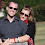## Friday, March 13, 2009

### Extension Methods for Picking an Item out of an IList

So, I've been at it again with the extension methods. Earlier this week, I had a problem where I was getting back an array of items from a webservice. This webservice builds these objects from data in a database. The table that stores these data uses GUIDs as their primary key and there's a clustered index on the id column. As a result, if you pick 2 items from the list, there's about a 50-50 chance they're in the right order.

I really need them to be in the right order, so I tried to add an order by clause. Unfortunately, this particular webservice isn't really all that good and there's no way to order the objects returned from the database. In this case, the fact is that I really just needed the most recent record anyhow.

I know what you're thinkin' and I thought the same thing. I could just sort the array and pop the first one off the top. The problem is that (and in this case it was definitely not a problem) sorting takes O(n log n) time and it's pretty easy to get a max or a min in O(n) time. I wrote a quick, "get the most recent in linear time" loop to get the element I wanted, and it occurred to me that I found another good extension method.

The problem was, GetMostRecent isn't really all that useful because I could very wall want, GetOldest, GetFirstAlphabetically, GetHottest, etc. Instead, I implemented GetBestFit and I provided several overloads for passing comparison logic in.

The first implementation I had took a Func<T, T, bool> as the comparator; however, I later found the Comparison<T> class which became a pretty decent wrapper for the Func<T, T, bool> parameter. I thought about getting rid of it altogether, but I still like the syntax of the former. Here are those two extension methods:
`public static T GetBestFit<T>(this IList<T> list, Func<T, T, bool> rule){    Comparison<T> c = new Comparison<T>((x, y) => (rule(x, y)) ? 1 : -1);    return list.GetBestFit(c);}public static T GetBestFit<T>(this IList<T> list, Comparison<T> comparison){    if (list == null || list.Count == 0)        throw new ArgumentException("You cannot get the best fit from an empty list!");    T currentFit = list;    for (int i = 1; i < list.Count; i++)        if (comparison(currentFit, list[i]) < 0)            currentFit = list[i];    return currentFit;}`
Then I realized that some objects already come with comparison information (id est, IComparable) and those objects deserve to have a GetBestFit that doesn't require you to write any comparison evaluators.
`public static T GetBestFit<T>(this IList<T> list)    where T : IComparable<T>{    if (list == null || list.Count == 0)        throw new ArgumentException("You cannot get the best fit from an empty list!");    T currentFit = list;    for (int i = 1; i < list.Count; i++)        if (currentFit.CompareTo(list[i]) < 0)            currentFit = list[i];    return currentFit;}`
Finally, I I thought that there are already classes responsible for comparing two objects for the sake of sorting and that getting the best fit is really just like sorting the list and taking the last item. I'm not really fixed on that one yet, but when you look at the way a comparer works and the way the sorting algorithm uses it, the "winner" always ends up at the end of the list. Anyhow, here's the IComparer implementation:
`public static T GetBestFit<T>(this IList<T> list, IComparer<T> comparer){    if (list == null || list.Count == 0)        throw new ArgumentException("You cannot get the best fit from an empty list!");    T currentFit = list;    for (int i = 1; i < list.Count; i++)        if (comparer.Compare(currentFit, list[i]) < 0)            currentFit = list[i];    return currentFit;}`
I wrote a full set of test cases using NUnit, but I'll spare you having to read those. However, it seems like some implementation information might be handy, so here are a few examples. I also wrote several IComparers, but only listed one in the current post for the sake of example.

I wrote a very basic person class to use with various comparisons:
`public class Person : IComparable<Person>{    public Person(string name, DateTime birthdate)    {        Name = name;        Birthdate = birthdate;    }    public string Name { get; set; }    public DateTime Birthdate { get; set; }    public int Age    {        get        {            return DateTime.MinValue.Add(DateTime.Now.Subtract(Birthdate)).Year - 1;        }    }    public int CompareTo(Person other)    {        return this.Age.CompareTo(other.Age);    }    public class AgeComparer : IComparer<Person>    {        public int Compare(Person x, Person y)        {            if (x.Age == y.Age) return 0;            return (x.Age > y.Age) ? 1 : -1;        }    }}`
I built a list of Persons and tested each extension with several different comparisons. Here are some syntax samples:
`// uses IComparable.CompareTolist.GetBestFit()// the rest of the tests used these variables, you know// just in case you want to run this codePerson oldest, first;// uses an ICompareroldest = list.GetBestFit(new Person.AgeComparer());first = list.GetBestFit(new Person.ReverseAlphabeticalComparer());// uses a Comparisonoldest = list.GetBestFit(new Comparison<Person>((x, y) => x.Age.CompareTo(y.Age)));first = list.GetBestFit(new Comparison<Person>((x, y) => y.Name.CompareTo(x.Name)));// uses a Func<T, T, bool> ruleoldest = list.GetBestFit((x, y) => x.Age > y.Age);first = list.GetBestFit((x, y) => x.Name.CompareTo(y.Name) < 0);`

1.2.